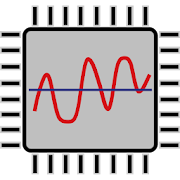# E & E Engineering. Free.

## Electrical and Electronics Engineering. Free version

4.52 TOOLS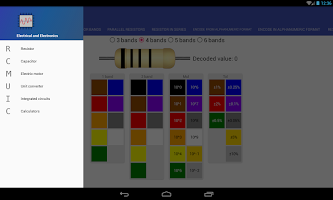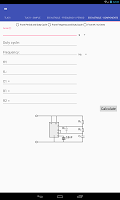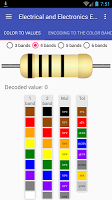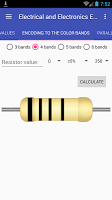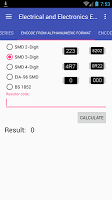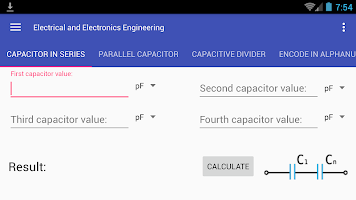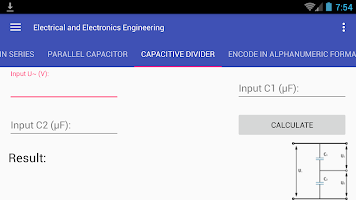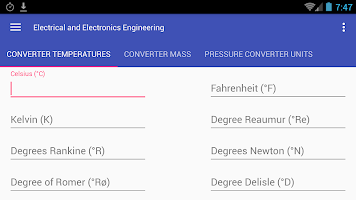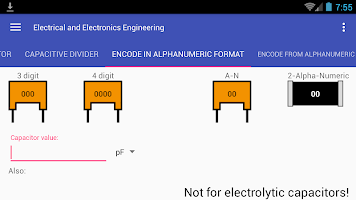A powerful application which contains useful for radio Amateurs, electrical engineering and the people related professions functionality. Will allow you to quickly perform the necessary conversions and calculations with minimal downtime.
Perfect for students.

In the program:
Resistors:
✔ Resistor color code calculator (value to color and color to value, 3-6 bands)
✔ Calculator for parallel resistors and resistor in series
✔ SMD resistor code calculator (Alpha-Numeric format, SMD 2, 3, 4 digit, EIA-96 and BS 1852)
✔ Calculator for resistive divider
✔ Led resistor calculator

Capacitors:
✔ Calculator for parallel and series capacitors
✔ Capacitive divider
✔ Capacitor color codes (Alpha-Numeric format)
✔ Capacitor reactance

Motor:
✔ Calculator for run and start motor capacitors (three-phase motor in single-phase)
✔ Calculation of the nominal motor current
✔ Active power consumed from network
✔ Nominal torque on the motor shaft calculator

Ohms law & etc.:
✔ Power calculation
✔ Voltage calculation
✔ Current calculation
✔ Power dissipated in the load (resistor)
✔ Frequency converter (frequency, period, wavelength)
✔ RC filter (component value -> frequency and frequency -> component value)
✔ LC circuit (frequency, capacitance and inductance)

PinOuts:
✔ USB port
✔ Parallel port
✔ Serial port (RS232)
✔ Ethernet (RJ45)

Conductor:
✔ Wire diameter ↔ Cross-sectional area converter

Unit converter:
✔ Pressure converter
✔ Mass converter
✔ Temperatures converter
✔ Energy and work
✔ Decibels at times

Integrated circuits: (Only in a Pro version)
✔ LM317 - voltage and current
✔ TL431 (two options)
✔ 555 (NE555 and clones) AStable, Monostable (frequency, period, components values)

### Details and Recent Ratings

Size: 3 MB
Version: 1.9 by D.I.K.S
Updated: 15 August 2018 (1131 days ago)
Released: 05 July 2016
Installations: more than 5 000
5 Stars: 67
4 Stars: 13
3 Stars: 6
2 Stars: 1
1 Star: 4

### Similar apps

Android Game Categories
Android App Categories